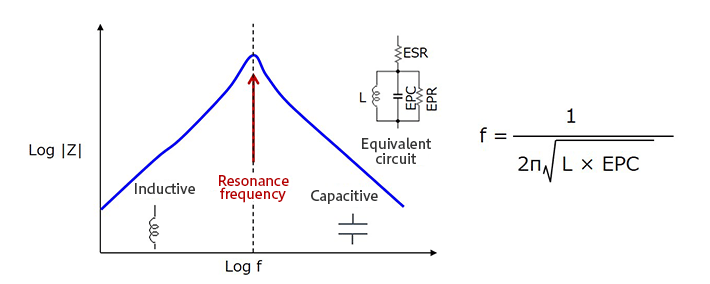# Frequency-Impedance Characteristics of Inductors and Determination of Inductor’s Resonance Frequency

2019.03.22

・An inductor exhibits an inductive characteristic (the impedance increases as the frequency rises) up to a resonance frequency.

・Beyond the resonance frequency, the inductor exhibits a capacitive characteristic (the impedance decreases as the frequency rises).

・At frequencies higher than the resonance frequency, the inductor does not function as an inductor.

・When the inductance L is small, the resonance frequency of the inductor is high.

・The impedance of an inductor at the resonance point is limited by parasitic resistance components.

## Understanding the Frequency Characteristics of Inductors

Before beginning an explanation of noise countermeasures using specific inductors, we first briefly review the frequency characteristics of inductors, similarly to the article explaining “Dealing with Noise Using Capacitors”.

An inductor (coil) has the following basic characteristics, which is said to be an inductive reactance.

① An inductor passes a direct current with essentially no change.
② An inductor acts to impede an alternating current.
③ The higher the frequency, the harder it is for an alternating current to flow.

The following is a schematic graph indicating the frequency-impedance characteristic of an inductor.In an ideal inductor, the impedance rises linearly with rising frequency, but in actuality, as indicated by the equivalent circuit, a parasitic capacitance EPC is present in parallel with the inductor, and so a self-resonance phenomenon occurs.

Hence up to the resonance frequency, the inductive characteristic inherent in the inductor (in which the impedance increases as the frequency rises) is exhibited, but beyond the resonance frequency, the influence of the parasitic capacitance becomes dominant, and a capacitive characteristic (in which the impedance decreases as the frequency rises) is exhibited. In other words, in the frequency region above the resonance frequency, the device no longer functions as an inductor.

The resonance frequency of an inductor can be found from the above equation. The equation is the same as the equation for the resonance frequency of a capacitor, the only difference being whether a term as the subject is capacitance or an inductance. As is clear from the equation, as the inductance L decreases, the resonance frequency rises.

Parasitic components of an inductor include, in addition to the electrostatic parasitic capacitance EPC, the resistance component of the inductor windings ESR (equivalent series resistance), and an EPR (equivalent parallel resistance) in parallel with the electrostatic capacitance. The resistance components limit the impedance at the resonance point.